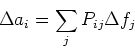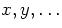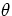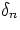Next: From Pixel to Physical Up: Sky Images Previous: Pixel Regularization

### 4.2.2.2 Transforming to Projected Sky Coordinate

The coordinate system of the FITS file data array, will, in general, be determined by the geometry of the instrumentation; consequently, the direction of the axes in the FITS file will need to be transformed to a coordinate system more natural to the physical system being measured. The initial FITS paper (Wells, Greisen, and Harten 1981) specifies use of the CROTAn keywords for describing how the axes of the FITS array are rotated to yield physical coordinates but does not define precisely how they are to be used. AIPS uses the CROTA2 keyword to represent the angle, in decimal degrees, of the declination or latitude axis with respect to the 2 axis of the data array, measured in the counterclockwise direction. AIPS does not use the CROTA1 keyword and assumes a default value of zero for the CROTA2 keyword. The CROTAn keywords are necessary only when the physical coordinates are rotated relative to the array coordinates. These AIPS conventions were endorsed at the Charlottesville meeting, have been widely used, and will be generally understood. However, some individual FITS files may define the CROTAn differently. Check the comments.

The CROTAn keywords cannot be applied in a straightforward way to more than two dimensions or to cases where the axes are not orthogonal. GC define the more general pixel coordinate (PC) matrix P to transform between the FITS array axes fj and axes in the direction of the physical coordinate system but on the array scale ai,(13)

where thesignify that positions are with respect to the reference point. Keywords are used to specify the elements of the PC matrix:

PCiiijjj (floating) is an multi-indexed keyword giving the value of the pixel coordinate matrix element Pij in equation 4.1.

The PC matrix technique permits considerable flexibility in transforming between axes, including axes that are not orthogonal. Some of this flexibility may be better left unused. In particular, it is possible to transpose the axes when going from the pixel axes () to the physical axes (). Suppose the first two axes are transposed, so that axis 2 in physical space corresponds to axis 1 in pixel space and vice versa. The most rapidly varying axis is axis 1 (i in pixel space), described by the values of NAXIS1 and CRPIX1. The corresponding physical axis is the one (y) described by CTYPE2, CRVAL2, and CDELT2. CTYPE1 describes the first axis in physical space, which is now not the most rapidly varying. If these concepts take some time to think through here, imagine trying to understand them in a FITS file. Such complications can be avoided if the tranformation is defined such that the diagonal terms of the PC matrix predominate. Another potential application of the PC matrix that is best unused is the ability to reverse the direction of the axis. GC strongly recommend that the elements of the PC matrix should be positive. Use the CDELTn keywords to reverse axis directions.

Because the AIPS convention has been widely used, new software that uses the PC matrix will still need to interpret files using the AIPS conventions. Also, until FITS reading software routinely includes support for the PC matrix, FITS files where the PC matrix represents a rotation equivalent to that of the old CROTAn method should also include the CROTAn keyword as well as the PC matrix. Ifis the latitude angle represented by CROTAn in the AIPS convention, andis the value of CDELTn, then

 P11 = cos(4.2) P12 = - (2 /1) sin(4.3) P21 = (1 /2) sin(4.4) P22 = cos(4.5)
It is always possible to express the CROTAn rotation in the PC matrix form. However, not all of the more general rotations described by the PC matrix can be described using CROTAn. When the coordinate transformation follows the AIPS convention, the P() relation can be inverted to yield two separate and equivalent expressions for:= tan -1{-[(1 /2) (P12 / P22)]} (4.6) =tan-1[(2 /1)(P21 / P11)] (4.7)

For a coordinate transformation following the AIPS convention, both expressions give the same value. For more general tranformations, both should be computed. If the difference between the two values ofobtained is within the expected precision, use the average (to reduce the round-off errors). If the difference is larger, then the coordinate axes are not orthogonal and the CROTAn description cannot be used (HW).Next: From Pixel to Physical Up: Sky Images Previous: Pixel Regularization